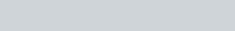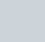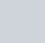reaction 2K+Br2->2K+ + 2Br-, chemistry homework help
1. In the following reaction, which species is reduced?

2K + Br2 →2K+ + 2Br

1. Arrange the following in order of increasing oxidation number of the sulfur atom1. The oxidation number of the sulfur atom in the SO 2/4– ion is

4 .A half-reactioninvolves a change in the oxidation state of an element.always contains H2O molecules.always contains H+ ions.All of the above

1. In the following reaction, which is the oxidizing agent? AgNO2+ Cl2 + 2KOH → AgNO3 + 2KCl + H2O
2. What are the oxidation states (in increasing order) of the element that undergoes disproportionation in the following reaction:

Cl2 + H2O → HCl + HOC

Which reaction is a redox reaction?Al2O3 + 6HCl → 2AlCl3+ 3H2O2HCO3 → CO2+ CO 2/3 – + H2OSiBr4 +3H2O → H2SiO3 + 4HBrH2O + PbO2 → NaOH + KCl → KClO + NaPb(OH)

6.

Which answer contains the correct information about the following reaction:

2Pb(NO3)2 → 2PbO + 4NO2– + O2This reaction is a decomposition reaction and not a redox reaction.This reaction is a redox reaction in which the lead is reduced and the oxygen is oxidized.This reaction is a disproportionation reaction.This reaction is a redox reaction in which the nitrogen is reduced and the oxygen is oxidized